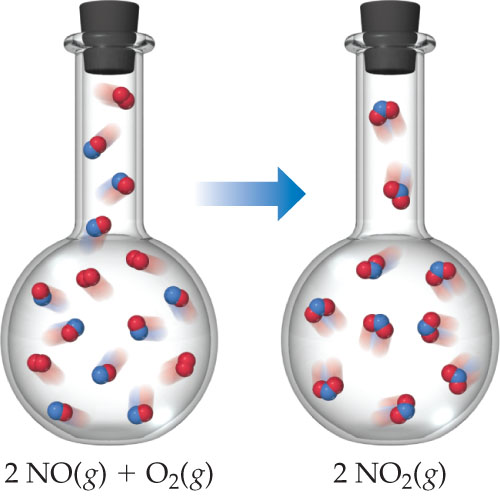Chemistry Practice Problems Entropy Practice Problems Solution: Entropy decreases when NO(g) is oxidized by O2(g) ...

# Solution: Entropy decreases when NO(g) is oxidized by O2(g) to NO2(g).What major factor leads to a decrease in entropy as the reaction shown takes place?

###### ProblemEntropy decreases when NO(g) is oxidized by O2(g) to NO2(g).

What major factor leads to a decrease in entropy as the reaction shown takes place?

###### Solution

We’re being asked to explain what factor leads to the decrease in entropy of the reaction:

2 NO(g) +   O2(g)   → 2 NO2(g)

Recall that entropy is the degree of chaos or disorder of a system and that it depends on the following:View Complete Written Solution

Entropy

Entropy

#### Q. The diagram shows the variation in entropy with temperature for a substance that is a gas at the highest temperature shown.Why is the entropy change f...

Solved • Thu Jan 03 2019 09:44:55 GMT-0500 (EST)

Entropy

#### Q. How does the entropy of the system change when a solid melts, a gas liquefies, a solid sublimes?

Solved • Thu Jan 03 2019 09:44:55 GMT-0500 (EST)

Entropy

#### Q. Estimate whether the entropy of the system increases or decreases during each process.(a) photodissociation of O2 (g),(b) formation of ozone from oxyg...

Solved • Thu Jan 03 2019 09:44:46 GMT-0500 (EST)

Entropy

#### Q. For which of the following processes does the entropy of the system increase?(a) the ripening of a banana,(b) dissolution of sugar in a cup of hot cof...

Solved • Thu Jan 03 2019 09:44:17 GMT-0500 (EST)